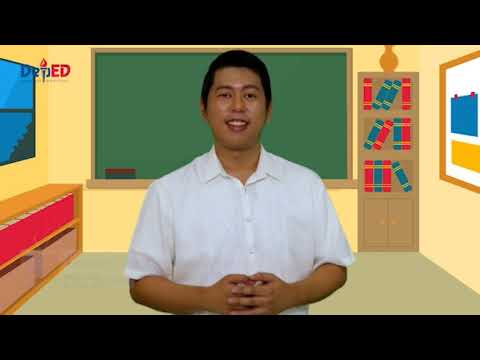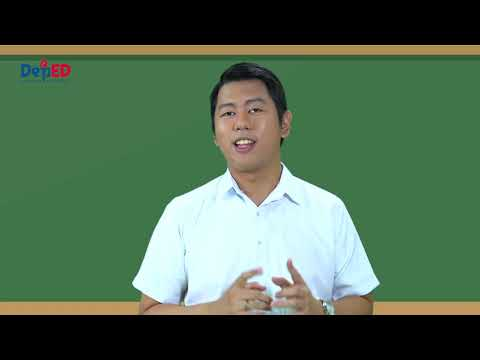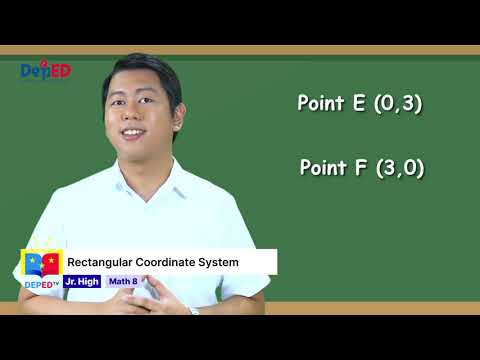### Grade 8 Math Q1 Ep1: Factoring Polynomials

Grade 8 Math Quarter 1 Episode 1: Factoring Polynomials Teacher: Joshua Salazar### Grade 8 Math Q1 Ep2: Factoring Special Products

Grade 8 Math Quarter 1 Episode 2: Factoring Special Products Teacher: Joshua Salazar### Grade 8 Math Q1 Ep3: Factoring Perfect Square and General Trinomials

Grade 8 Math Quarter 1 Episode 3 : Factoring Perfect Square and General Trinomials Teacher : Joshua Salazar### Grade 8 Math Q1 Ep4: Solving Problems Involving Factors of Polynomials

Grade 8 Math Quarter 1 Episode 4 : Solving Problems Involving Factors of Polynomials Teacher : Joshua Salazar### Grade 8 Math Q1 Ep5: Rational Algebraic Expressions

Grade 8 Math Quarter 1 Episode 5: Rational Algebraic Expressions Teacher: Joshua Salazar### Grade 8 Math Q1 Ep 6 Simplifying Rational Algebraic Expressions

Grade 8 Math Quarter 1 Episode 6 : Simplifying Rational Algebraic Expressions Teacher : Joshua P. Salazar### Grade 8 Math Q1 Ep 7 Multiplication and Division on Rational Algebraic Expression

Grade 8 Math Quarter 1 Episode 7 : Multiplication and Division on Rational Algebraic Expression Teacher : Joshua Salazar### Grade 8 Math Q1 Ep 8 Adding and Subtracting Rational Algebraic Expressions

Grade 8 Math Quarter 1 Episode 8 : Adding and Subtracting Rational Algebraic Expressions Teacher : Joshua Salazar### Grade 8 Math Q1 Ep9: Rectangular Coordinate System

Grade 8 Math Quarter 1 Episode 9: Rectangular Coordinate System Teacher: Joshua Salazar### Grade 8 Math Q1 Ep10: Linear Equation

Grade 8 Math Quarter 1 Episode 10: Linear Equation Teacher: Joshua Salazar### Grade 8 Math Q1 Ep 11 Slope of the Line

Grade 8 Math Quarter 1 Episode 11 : Slope of the Line Teacher : Joshua P. Salazar### Grade 8 Math Q1 Ep 12 Graphing Linear Equations

Grade 8 Math Quarter 1 Episode 12 : Graphing Linear Equations Teacher : Joshua P. Salazar### Grade 8 Math Q1 Ep 13 Finding the Equation of the Line

Grade 8 Math Quarter 1 Episode 13: Finding the Equation of the Line Teacher: Sir Joshua Salazar### Grade 8 Math Q1 Ep 14 Solving Problems Involving Linear Equations in Two Variables

Grade 8 Mathematics Quarter 1 Episode 14: Solving Problems Involving Linear Equations in Two Variables Teacher: Sir Joshua Salazar### Grade 8 Math Q1 Ep 15 Systems of Linear Equations in Two Variables

Grade 8 Math Quarter 1 Episode 15 Systems of Linear Equations in Two Variables Teacher: Sir Joshua Salazar### Grade 8 Math Q1 Ep 16 Graphing Systems of Linear Equations in Two Variables

Grade 8 Math Quarter 1 Episode 16 : Graphing Systems of Linear Equations in Two Variables Teacher : Joshua P. Salazar### Grade 8 Math Q1 Ep 17 Solving Systems of Linear Equations by Substitution

Grade 8 Math Quarter 1 Episode 17 : Solving Systems of Linear Equations by Substitution Teacher : Joshua P. Salazar### Grade 8 Math Q1 Ep 18 Solving Systems of Linear Equations by Elimination

Grade 8 Math Quarter 1 Episode 18 : Solving Systems of Linear Equations by Elimination Teacher : Joshua P. Salazar